### Home > CALC > Chapter 8 > Lesson 8.1.5 > Problem8-52

8-52.
1. Let y = 3x + 1, y = 0, x = 0, and x = 2. Find the volume of the region revolved about each of the lines below. Homework Help ✎

1. x-axis

2. y-axis

3. y = −1

4. Explain why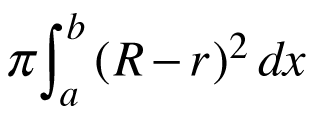is the incorrect set-up for a washer given.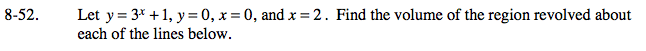Be sure to sketch the region before beginning.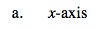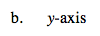If y = 3x + 1, then x = log3(y − 1).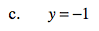Notice, both the outer and the inner radii are 1 unit longer than in part (a).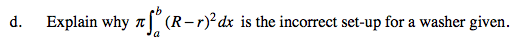True or False? R2r2 = (Rr)2